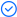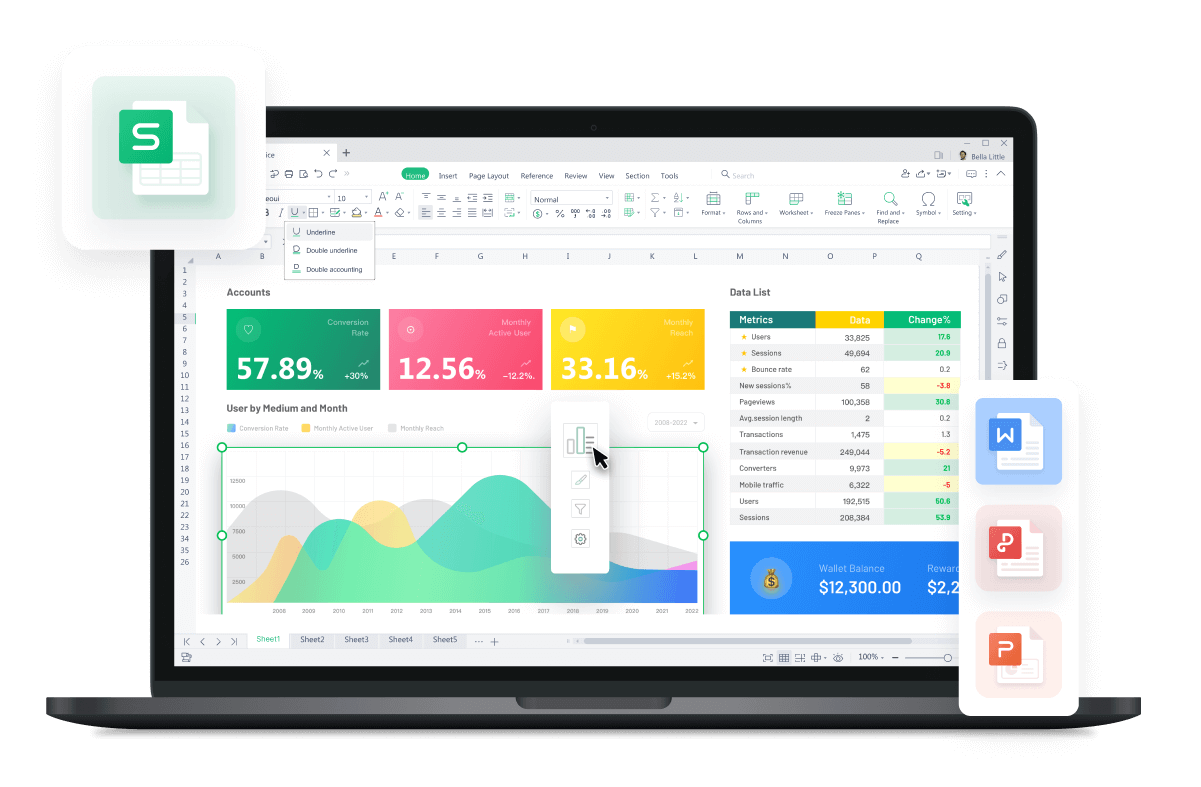WPS Office

Free All-in-One Office Suite with PDF EditorRead, edit, and convert PDFs with the powerful PDF toolkit.Microsoft-like interface, easy to use.

Windows • MacOS • Linux • iOS • Android# Recalculate the table formulas in the Manual Recalculation mode

Uploaded time: November 22, 2021 Difficulty Beginner

# Recalculate the table formulas in the Manual Recalculation modeRecalculate the table formulas in the Manual Recalculation mode

Today, let me introduce you to a lesser-used function, Manual Recalculation.

Take this document as an example. Now, the cells have formulas. When we modify the source data, the values in the table will also change automatically.That is because the table turns on the automatic calculation by default.

When we need to process a particularly large amount of data in the worksheet, if we still choose automatic calculation, then every time we change the source data, the table data processing speed will slow down. At this point, we can switch to Manual Recalculationmode.

First, we need to click the Menu drop-down menu and click Options, then select the Calculation option to check theManual option. When we modify the source data, the values of the table are no longer calculated automatically.

So how can we calculate manually in the Manual mode? Click the Formulastab and the Calculate Sheet button.Then, the formula of the table will calculate the correct result.

We can also recalculate the formula results with shortcut F9.

To be office excel advanced, you could learn how to use WPS Office Spreadsheet online in WPS Academy.